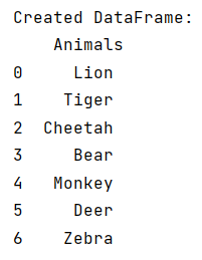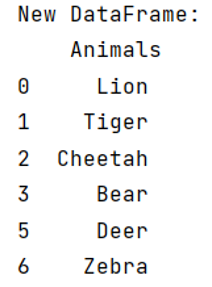# How to search for 'does-not-contain' on a DataFrame in pandas?

Given a Pandas DataFrame, we have to search for 'does-not-contain' on it.
Submitted by Pranit Sharma, on June 01, 2022

Searching and filtering in pandas is a complex task, however the use of loc() made searching and filtering based on certain conditions much easier for the analysts to analyse the data without any difficulties.

Here, we are going to learn how to search for 'does-not-contain' on a DataFrame? By 'does-not-contain', we mean that a particular object will not be present in the new DataFrame.

This can be done with the help of invert (~) operator, it acts as a not operator when the values are True or False. If the value is True for the entire column, new DataFrame will be same as original but if the values is False, it will eliminate that particular string from the new DataFrame.

To work with pandas, we need to import pandas package first, below is the syntax:

```import pandas as pd
```

Let us understand with the help of an example,

```# Importing pandas package
import pandas as pd

# Creating a DataFrame
df =pd.DataFrame(['Lion','Tiger','Cheetah','Bear','Monkey','Deer','Zebra'],
columns=['Animals'])

# Display Created DataFrame

print("Created DataFrame:\n",df,"\n")
```

Output:Now, we will see how to search for 'does-not-contain' using invert(~) operator.

```# Searching in the column Animals where
# it contains Monkey
new_df = df[~df['Animals'].str.contains('Monkey', na=False)]

# Display new_df
print("New DataFrame:\n",new_df)
```

Output:Preparation

What's New

Top Interview Coding Problems/Challenges!